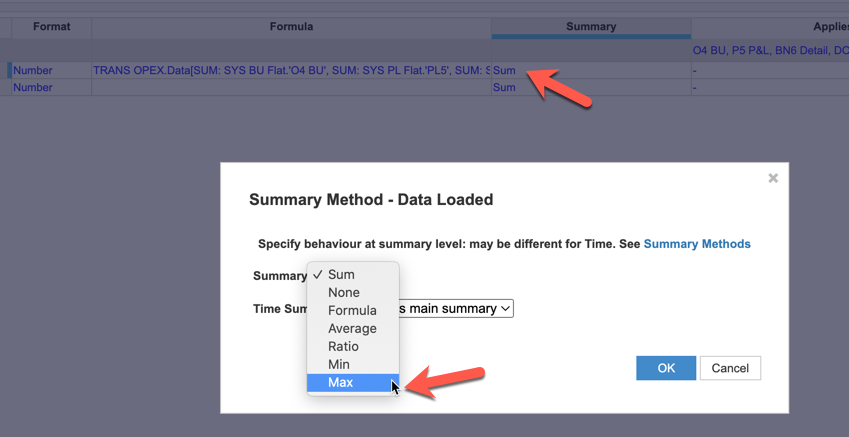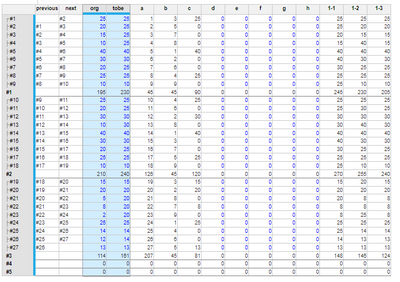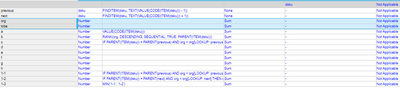# How to calculate within the list items to get the maximun of the items from the first to current?Hi everybody!

Need help to do the calcution within the list.

Here is a list with items(#1,#2,#3,...), and properties of Input and Output.

The Output is supposed to be the maximun value of the input from #1 to the current item as below table.

 Name Input Output ※How to calculate the Output #1 25 25 =MAX(#1:#1) #2 20 25 =MAX(#1:#2) #3 30 30 =MAX(#1:#3) #4 10 30 =MAX(#1:#4) #5 40 40 =MAX(#1:#5) #6 30 40 =MAX(#1:#6) #7 20 40 =MAX(#1:#7) #8 25 40 =MAX(#1:#8) #9 10 40 =MAX(#1:#9)
Tagged:

•This looks fun...Let me ask you this, how many list members are you talking about, the 1-9 in your example?

•#1~9 is just an example.^^
Actually the list have about 500,000 members and parent of about 100,000 members, e.g. 1 parent with 1~20 children.
And the calculation just need to do within the members under the same parent, as the example above you could image that the #1~9 have the same parent.
Please let me know if you need any further explanation on this.
•And you just want the Max number at the parent level?  Have you looked into the summary method of Max?•I want the max value of the member which is within #1~current as example above which is used to adjust the input value.
•Here is another example.

If the List item have values like,

10, 15, 5, 20, 10, 30, 20, 25, 5

Then the results I want is,

10, 15, 15, 20, 20, 30, 30, 25, 5

These list items have the same parent but summary is no need and the calculation only has to be done on the child level.

Best regards!

•Can you do me/us a favor and show use a true sample of the List member as well as the code?  Is Time or Period part of it?

Rob

•We are still confirming the feasibility of this requirement.

Here is the test module (no time dimension), 'org' is input data, 'tobe' is what we want to calculate, and others are my tentative calculations, you can ignore them.best regards,

Cecilia Ren